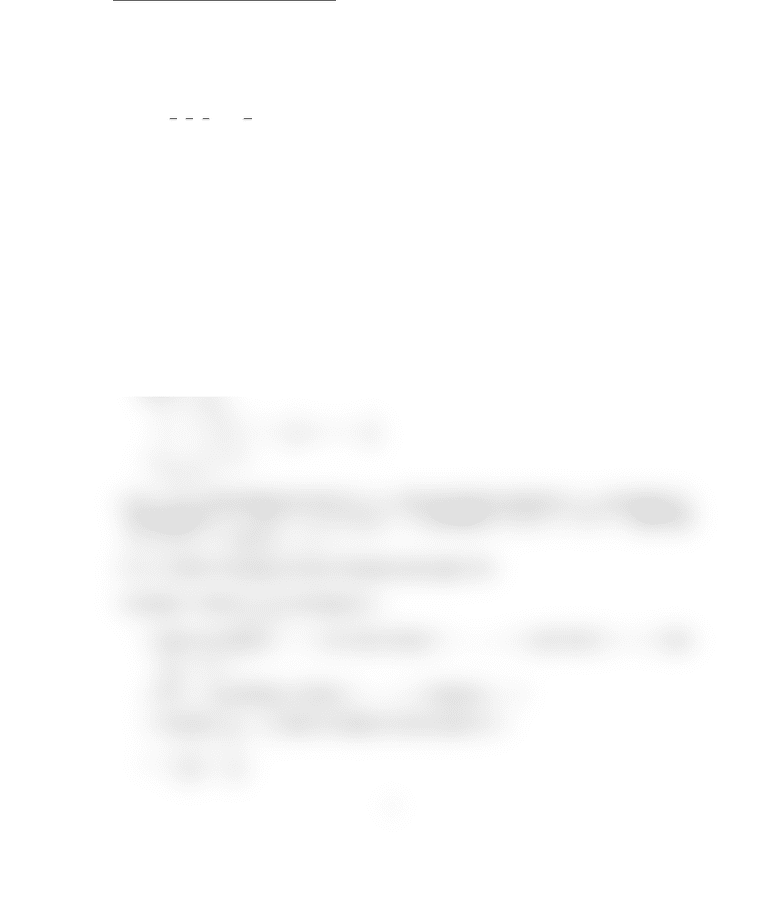Class Notes (1,100,000)
CA (620,000)
UTSC (30,000)
Mathematics (1,000)
MATA37H3 (100)
Lecture 14

# MATA37 Lecture 14: SequencesPremium

Department
Mathematics
Course Code
MATA37H3
Professor
Smith( G)
Lecture
14

This preview shows half of the first page. to view the full 2 pages of the document.MATA37 - Lecture 14 - Sequences
Sequences
See section 7.1 and 7.2
Idea: An inﬁnite sequence of real numbers is an inﬁnite order list of real numbers.
e.g. 1,1
2,1
3,1
4,..., 1
n, . . .
In general, a1, a2, . . . , an, . . .
An inﬁnite sequence is a real-valued function whose domain is N, denoted in one of the
following ways (see page 579):
{an}
n=1
{an}(no index listed means start at 1)
an=f(n)
The term anis called the general term.
Examples of sequences:
{n}
1,2,3,4, . . .
1 + 1
2n
n=1
a1= 1 + 1
2, a2= 1 + 1
22, a3= 1 + 1
23
an= 1 + (1)n
0,2,0,2, . . .
Let lR(lrepresenting the limit of {an}). We say that the sqeuence {an}converges to l
(symbolically, anlas n→ ∞, or lim
n→∞
an=l), provided lR, > 0,N > 0such that
nN, if n>N, then |anl|< 
If {an}doesn’t converge, we say it diverges (see page 579).
Example 1: Show 1
nconverges to 0.
Given an arbitrary  > 0, we must choose N=>0, such that if n > N , then
1
n0
< 
Let  > 0be arbitrary. Choose N=>0. Suppose n>N
Consider
1
n0
. Want a multiple of some power of N.
=
1
n
=1
n
1
###### You're Reading a Preview

Unlock to view full version

Subscribers Only